# AP Calculus AB Practice Test 39

### Test Information9 questions27 minutes

Calculator Allowed

1. The area bounded by the curve x = 3y - y2 and the line x = - y is represented by

2. The region bounded by y = ex, y = 1, and x = 2 is rotated about the x-axis. The volume of the solid generated is given by the integral:

3. A particle moves on a straight line so that its velocity at time t is given by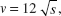where s is its distance from the origin. If s = 1 when t = 0, then, when t = 1, s equals

4.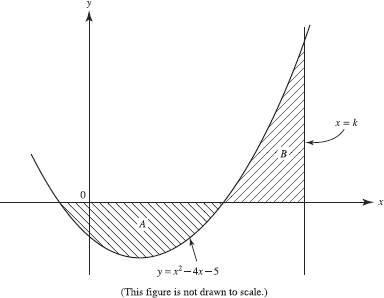The sketch shows the graphs of f (x) = x2 - 4x - 5 and the line x = k. The regions labeled A and B have equal areas if k =

5. Bacteria in a culture increase at a rate proportional to the number present. An initialpopulation of 200 triples in 10 hours. If this pattern of increase continues unabated,then the approximate number of bacteria after 1 full day is

6. When the substitution x = 2t - 1 is used, the definite integral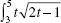dt may be expressed in the form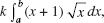where {k, a, b} =

7. The curve defined by x3 + xy - y2 = 10 has a vertical tangent line when x =

8. Use the graph of f shown on [0,7] for Question below. Let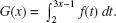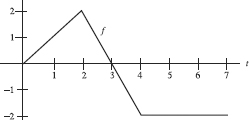G (1) is

9. Use the graph of f shown on [0,7] for Question below. LetG has a local maximum at x =